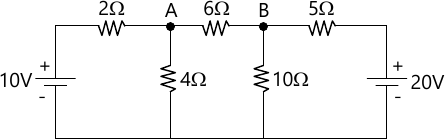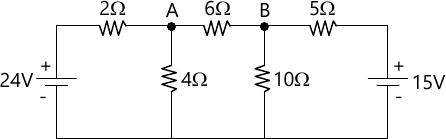MORE IN Basic Electrical Engineering (BEE)
SPPU First Year Engineering (Semester 1)
Basic Electrical Engineering (BEE)
June 2015
Total marks: --
Total time: --
INSTRUCTIONS
(1) Assume appropriate data and state your reasons
(2) Marks are given to the right of every question
(3) Draw neat diagrams wherever necessary

Answer any one question from Q1 and Q2
1 (a) If a coil of 150 turns is linked with a flux of 0.01 Wb when carrying a current of 10 A, then calculate the induced emf.
i) if this current is uniformly reversed in 0.1 second.
(ii) if this current is interrupted in 0.05 second.
6 M
1 (b) Define insulation resistance and obtain an expression for insulation resistance of a single core cable.
6 M

2 (a) Explain what do you mean by statically induced EMF and dynamically induced EMF
6 M
2 (b) A coil of insulated copper wire has a resistance of 150 Ω at 20°C. When the coil is connected across a 240 V supply, the current after several hours is 1.25 A. Calculate the temperature of the coil, assuming the temp. co-efficient of resistance of 20°C to be 0.0039 per °C.
6 M

Answer any one question from Q3 and Q4
3 (a) Derive an EMF equation of 1-ph transformer.
6 M
3 (b) Derive an expression for instantaneous current and power consumed when voltage of V = Vm sin ( t) is applied to pure inductance alone. Also draw the phasor diagram.
6 M

4 (a) A single phase 4 kVA transformer has 400 turns on its primary and 1000 secondary turns. The net cross-sectional area of the core is 60 cm2. When the primary winding is connected to 500 V, 50 Hz supply, calculate:
i) the max. value of flux density in the core
(ii) the voltage induced in the secondary winding and
(iii) the secondary full load current.
6 M
4 (b) The expression of the alternating current is given by i = 5.48 sin Ωt:
Calculate:
(i) the average value
(ii) RMS value of the current
(iii) power consumed if the current is passed through a resistance of 10 Ω.
6 M

Answer any one question from Q5 and Q6
5 (a) What is admittance of an AC circuit ? What are its two components? State units of these quantities. How the admittance is expressed in rectangular and polar form?
6 M
5 (b) An impedance Z1 = (100 + j0) Ω is connected in series with another impedance Z2 = (50 + j80) . The circuit is connected to a single phase 230 V, 50 Hz supply. Calculate:
i) current drawn by the circuit
(ii) power consumed by whole circuit
(iii) circuit power factor.
7 M

6 (a) Draw and explain phasor diagram of an RLC series circuit, When :
i) XC > XL.
ii) XC < XL
iii) XC = XL
7 M
6 (b) A delta connected balanced load across a 400 V 3-phase supply consist of three identical impedances, each equal to (15+j12) Ω Find the line current, active power and reactive power.
6 M

Answer any one question from Q7 and Q8
7 (a) State and explain Superposition Theorem.
6 M
7 (b) Using Kirchhoff's Law, determine the current flowing through 6 &Omelga; resistance.7 M

8 (a) Derive the expressions to convert delta connected resistances into equivalent star circuit.
6 M
8 (b) Using Thevenin's Theorem, determine the value of current flowing through 6 Ω resistance.7 M

More question papers from Basic Electrical Engineering (BEE)